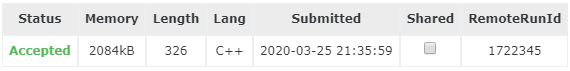# LightOJ 1274 Beating the Dataset 组合/期望DP

Beating the Dataset LightOJ - 1274

$n$ 个文件，每个文件里面包含 $YES$ 或者 $NO$，前者 3 bytes，后者 2 bytes，并且知道这些文件的总大小 $s$

$\frac{(2xy\cdot(x+y-1)!+x\cdot(x+y-1)!)}{(x+y)!}=\frac{2xy+x}{x+y}$

#include<iostream>
#include<cstdio>
//#define WINE
using namespace std;
int T,iCase,n,s,x,y;
int main(){
#ifdef WINE
freopen("data.in","r",stdin);
#endif
scanf("%d",&T);
while(T--){
scanf("%d%d",&n,&s);
y=s-2*n;x=n-y;
printf("Case %d: %.8lf\n",++iCase,(2.0*x*y+x)/n);
}
return 0;
}©️2019 CSDN 皮肤主题: 深蓝海洋 设计师: CSDN官方博客